## 5.1  Designing Register Machines

To design a register machine, we must design its data paths (registers and operations) and the controller that sequences these operations. To illustrate the design of a simple register machine, let us examine Euclid's Algorithm, which is used to compute the greatest common divisor (GCD) of two integers. As we saw in section 1.2.5, Euclid's Algorithm can be carried out by an iterative process, as specified by the following procedure:

(define (gcd a b)
(if (= b 0)
a
(gcd b (remainder a b))))

A machine to carry out this algorithm must keep track of two numbers, a and b, so let us assume that these numbers are stored in two registers with those names. The basic operations required are testing whether the contents of register b is zero and computing the remainder of the contents of register a divided by the contents of register b. The remainder operation is a complex process, but assume for the moment that we have a primitive device that computes remainders. On each cycle of the GCD algorithm, the contents of register a must be replaced by the contents of register b, and the contents of b must be replaced by the remainder of the old contents of a divided by the old contents of b. It would be convenient if these replacements could be done simultaneously, but in our model of register machines we will assume that only one register can be assigned a new value at each step. To accomplish the replacements, our machine will use a third ``temporary'' register, which we call t. (First the remainder will be placed in t, then the contents of b will be placed in a, and finally the remainder stored in t will be placed in b.)

We can illustrate the registers and operations required for this machine by using the data-path diagram shown in figure 5.1. In this diagram, the registers (a, b, and t) are represented by rectangles. Each way to assign a value to a register is indicated by an arrow with an X behind the head, pointing from the source of data to the register. We can think of the X as a button that, when pushed, allows the value at the source to ``flow'' into the designated register. The label next to each button is the name we will use to refer to the button. The names are arbitrary, and can be chosen to have mnemonic value (for example, a<-b denotes pushing the button that assigns the contents of register b to register a). The source of data for a register can be another register (as in the a<-b assignment), an operation result (as in the t<-r assignment), or a constant (a built-in value that cannot be changed, represented in a data-path diagram by a triangle containing the constant).

An operation that computes a value from constants and the contents of registers is represented in a data-path diagram by a trapezoid containing a name for the operation. For example, the box marked rem in figure 5.1 represents an operation that computes the remainder of the contents of the registers a and b to which it is attached. Arrows (without buttons) point from the input registers and constants to the box, and arrows connect the operation's output value to registers. A test is represented by a circle containing a name for the test. For example, our GCD machine has an operation that tests whether the contents of register b is zero. A test also has arrows from its input registers and constants, but it has no output arrows; its value is used by the controller rather than by the data paths. Overall, the data-path diagram shows the registers and operations that are required for the machine and how they must be connected. If we view the arrows as wires and the X buttons as switches, the data-path diagram is very like the wiring diagram for a machine that could be constructed from electrical components.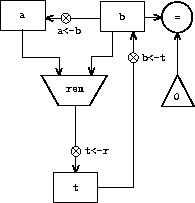In order for the data paths to actually compute GCDs, the buttons must be pushed in the correct sequence. We will describe this sequence in terms of a controller diagram, as illustrated in figure 5.2. The elements of the controller diagram indicate how the data-path components should be operated. The rectangular boxes in the controller diagram identify data-path buttons to be pushed, and the arrows describe the sequencing from one step to the next. The diamond in the diagram represents a decision. One of the two sequencing arrows will be followed, depending on the value of the data-path test identified in the diamond. We can interpret the controller in terms of a physical analogy: Think of the diagram as a maze in which a marble is rolling. When the marble rolls into a box, it pushes the data-path button that is named by the box. When the marble rolls into a decision node (such as the test for b = 0), it leaves the node on the path determined by the result of the indicated test. Taken together, the data paths and the controller completely describe a machine for computing GCDs. We start the controller (the rolling marble) at the place marked start, after placing numbers in registers a and b. When the controller reaches done, we will find the value of the GCD in register a.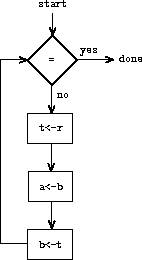Exercise 5.1.  Design a register machine to compute factorials using the iterative algorithm specified by the following procedure. Draw data-path and controller diagrams for this machine.

(define (factorial n)
(define (iter product counter)
(if (> counter n)
product
(iter (* counter product)
(+ counter 1))))
(iter 1 1))

### 5.1.1  A Language for Describing Register Machines

Data-path and controller diagrams are adequate for representing simple machines such as GCD, but they are unwieldy for describing large machines such as a Lisp interpreter. To make it possible to deal with complex machines, we will create a language that presents, in textual form, all the information given by the data-path and controller diagrams. We will start with a notation that directly mirrors the diagrams.

We define the data paths of a machine by describing the registers and the operations. To describe a register, we give it a name and specify the buttons that control assignment to it. We give each of these buttons a name and specify the source of the data that enters the register under the button's control. (The source is a register, a constant, or an operation.) To describe an operation, we give it a name and specify its inputs (registers or constants).

We define the controller of a machine as a sequence of instructions together with labels that identify entry points in the sequence. An instruction is one of the following:

• The name of a data-path button to push to assign a value to a register. (This corresponds to a box in the controller diagram.)

• A test instruction, that performs a specified test.

• A conditional branch (branch instruction) to a location indicated by a controller label, based on the result of the previous test. (The test and branch together correspond to a diamond in the controller diagram.) If the test is false, the controller should continue with the next instruction in the sequence. Otherwise, the controller should continue with the instruction after the label.

• An unconditional branch (goto instruction) naming a controller label at which to continue execution.

The machine starts at the beginning of the controller instruction sequence and stops when execution reaches the end of the sequence. Except when a branch changes the flow of control, instructions are executed in the order in which they are listed.

 (data-paths  (registers   ((name a)    (buttons ((name a<-b) (source (register b)))))   ((name b)    (buttons ((name b<-t) (source (register t)))))   ((name t)    (buttons ((name t<-r) (source (operation rem))))))  (operations   ((name rem)    (inputs (register a) (register b)))   ((name =)    (inputs (register b) (constant 0))))) (controller  test-b                           ; label    (test =)                       ; test    (branch (label gcd-done))      ; conditional branch    (t<-r)                         ; button push    (a<-b)                         ; button push    (b<-t)                         ; button push    (goto (label test-b))          ; unconditional branch  gcd-done)                        ; label

Figure 5.3 shows the GCD machine described in this way. This example only hints at the generality of these descriptions, since the GCD machine is a very simple case: Each register has only one button, and each button and test is used only once in the controller.

Unfortunately, it is difficult to read such a description. In order to understand the controller instructions we must constantly refer back to the definitions of the button names and the operation names, and to understand what the buttons do we may have to refer to the definitions of the operation names. We will thus transform our notation to combine the information from the data-path and controller descriptions so that we see it all together.

To obtain this form of description, we will replace the arbitrary button and operation names by the definitions of their behavior. That is, instead of saying (in the controller) ``Push button t<-r'' and separately saying (in the data paths) ``Button t<-r assigns the value of the rem operation to register t'' and ``The rem operation's inputs are the contents of registers a and b,'' we will say (in the controller) ``Push the button that assigns to register t the value of the rem operation on the contents of registers a and b.'' Similarly, instead of saying (in the controller) ``Perform the = test'' and separately saying (in the data paths) ``The = test operates on the contents of register b and the constant 0,'' we will say ``Perform the = test on the contents of register b and the constant 0.'' We will omit the data-path description, leaving only the controller sequence. Thus, the GCD machine is described as follows:

(controller
test-b
(test (op =) (reg b) (const 0))
(branch (label gcd-done))
(assign t (op rem) (reg a) (reg b))
(assign a (reg b))
(assign b (reg t))
(goto (label test-b))
gcd-done)

This form of description is easier to read than the kind illustrated in figure 5.3, but it also has disadvantages:

• It is more verbose for large machines, because complete descriptions of the data-path elements are repeated whenever the elements are mentioned in the controller instruction sequence. (This is not a problem in the GCD example, because each operation and button is used only once.) Moreover, repeating the data-path descriptions obscures the actual data-path structure of the machine; it is not obvious for a large machine how many registers, operations, and buttons there are and how they are interconnected.

• Because the controller instructions in a machine definition look like Lisp expressions, it is easy to forget that they are not arbitrary Lisp expressions. They can notate only legal machine operations. For example, operations can operate directly only on constants and the contents of registers, not on the results of other operations.

In spite of these disadvantages, we will use this register-machine language throughout this chapter, because we will be more concerned with understanding controllers than with understanding the elements and connections in data paths. We should keep in mind, however, that data-path design is crucial in designing real machines.

Exercise 5.2.  Use the register-machine language to describe the iterative factorial machine of exercise 5.1.

#### Actions

Let us modify the GCD machine so that we can type in the numbers whose GCD we want and get the answer printed at our terminal. We will not discuss how to make a machine that can read and print, but will assume (as we do when we use read and display in Scheme) that they are available as primitive operations.1

Read is like the operations we have been using in that it produces a value that can be stored in a register. But read does not take inputs from any registers; its value depends on something that happens outside the parts of the machine we are designing. We will allow our machine's operations to have such behavior, and thus will draw and notate the use of read just as we do any other operation that computes a value.

Print, on the other hand, differs from the operations we have been using in a fundamental way: It does not produce an output value to be stored in a register. Though it has an effect, this effect is not on a part of the machine we are designing. We will refer to this kind of operation as an action. We will represent an action in a data-path diagram just as we represent an operation that computes a value -- as a trapezoid that contains the name of the action. Arrows point to the action box from any inputs (registers or constants). We also associate a button with the action. Pushing the button makes the action happen. To make a controller push an action button we use a new kind of instruction called perform. Thus, the action of printing the contents of register a is represented in a controller sequence by the instruction

(perform (op print) (reg a))

Figure 5.4 shows the data paths and controller for the new GCD machine. Instead of having the machine stop after printing the answer, we have made it start over, so that it repeatedly reads a pair of numbers, computes their GCD, and prints the result. This structure is like the driver loops we used in the interpreters of chapter 4.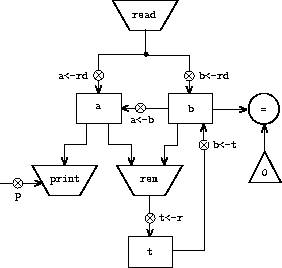(controller   gcd-loop     (assign a (op read))     (assign b (op read))   test-b     (test (op =) (reg b) (const 0))     (branch (label gcd-done))     (assign t (op rem) (reg a) (reg b))     (assign a (reg b))     (assign b (reg t))     (goto (label test-b))   gcd-done     (perform (op print) (reg a))     (goto (label gcd-loop)))

### 5.1.2  Abstraction in Machine Design

We will often define a machine to include ``primitive'' operations that are actually very complex. For example, in sections 5.4 and 5.5 we will treat Scheme's environment manipulations as primitive. Such abstraction is valuable because it allows us to ignore the details of parts of a machine so that we can concentrate on other aspects of the design. The fact that we have swept a lot of complexity under the rug, however, does not mean that a machine design is unrealistic. We can always replace the complex ``primitives'' by simpler primitive operations.

Consider the GCD machine. The machine has an instruction that computes the remainder of the contents of registers a and b and assigns the result to register t. If we want to construct the GCD machine without using a primitive remainder operation, we must specify how to compute remainders in terms of simpler operations, such as subtraction. Indeed, we can write a Scheme procedure that finds remainders in this way:

(define (remainder n d)
(if (< n d)
n
(remainder (- n d) d)))

We can thus replace the remainder operation in the GCD machine's data paths with a subtraction operation and a comparison test. Figure 5.5 shows the data paths and controller for the elaborated machine. The instruction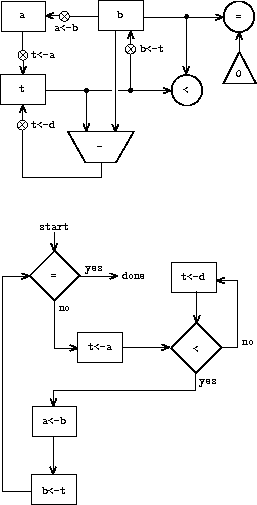(assign t (op rem) (reg a) (reg b))

in the GCD controller definition is replaced by a sequence of instructions that contains a loop, as shown in figure 5.6.

 (controller  test-b    (test (op =) (reg b) (const 0))    (branch (label gcd-done))    (assign t (reg a))  rem-loop    (test (op <) (reg t) (reg b))    (branch (label rem-done))    (assign t (op -) (reg t) (reg b))    (goto (label rem-loop))  rem-done    (assign a (reg b))    (assign b (reg t))    (goto (label test-b))  gcd-done)

Exercise 5.3.  Design a machine to compute square roots using Newton's method, as described in section 1.1.7:

(define (sqrt x)
(define (good-enough? guess)
(< (abs (- (square guess) x)) 0.001))
(define (improve guess)
(average guess (/ x guess)))
(define (sqrt-iter guess)
(if (good-enough? guess)
guess
(sqrt-iter (improve guess))))
(sqrt-iter 1.0))

Begin by assuming that good-enough? and improve operations are available as primitives. Then show how to expand these in terms of arithmetic operations. Describe each version of the sqrt machine design by drawing a data-path diagram and writing a controller definition in the register-machine language.

### 5.1.3  Subroutines

When designing a machine to perform a computation, we would often prefer to arrange for components to be shared by different parts of the computation rather than duplicate the components. Consider a machine that includes two GCD computations -- one that finds the GCD of the contents of registers a and b and one that finds the GCD of the contents of registers c and d. We might start by assuming we have a primitive gcd operation, then expand the two instances of gcd in terms of more primitive operations. Figure 5.7 shows just the GCD portions of the resulting machine's data paths, without showing how they connect to the rest of the machine. The figure also shows the corresponding portions of the machine's controller sequence.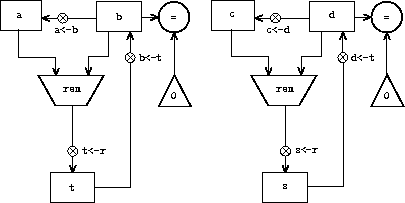gcd-1  (test (op =) (reg b) (const 0))  (branch (label after-gcd-1))  (assign t (op rem) (reg a) (reg b))  (assign a (reg b))  (assign b (reg t))  (goto (label gcd-1)) after-gcd-1gcd-2  (test (op =) (reg d) (const 0))  (branch (label after-gcd-2))  (assign s (op rem) (reg c) (reg d))  (assign c (reg d))  (assign d (reg s))  (goto (label gcd-2)) after-gcd-2

This machine has two remainder operation boxes and two boxes for testing equality. If the duplicated components are complicated, as is the remainder box, this will not be an economical way to build the machine. We can avoid duplicating the data-path components by using the same components for both GCD computations, provided that doing so will not affect the rest of the larger machine's computation. If the values in registers a and b are not needed by the time the controller gets to gcd-2 (or if these values can be moved to other registers for safekeeping), we can change the machine so that it uses registers a and b, rather than registers c and d, in computing the second GCD as well as the first. If we do this, we obtain the controller sequence shown in figure 5.8.

We have removed the duplicate data-path components (so that the data paths are again as in figure 5.1), but the controller now has two GCD sequences that differ only in their entry-point labels. It would be better to replace these two sequences by branches to a single sequence -- a gcd subroutine -- at the end of which we branch back to the correct place in the main instruction sequence. We can accomplish this as follows: Before branching to gcd, we place a distinguishing value (such as 0 or 1) into a special register, continue. At the end of the gcd subroutine we return either to after-gcd-1 or to after-gcd-2, depending on the value of the continue register. Figure 5.9 shows the relevant portion of the resulting controller sequence, which includes only a single copy of the gcd instructions.

 gcd-1  (test (op =) (reg b) (const 0))  (branch (label after-gcd-1))  (assign t (op rem) (reg a) (reg b))  (assign a (reg b))  (assign b (reg t))  (goto (label gcd-1)) after-gcd-1gcd-2  (test (op =) (reg b) (const 0))  (branch (label after-gcd-2))  (assign t (op rem) (reg a) (reg b))  (assign a (reg b))  (assign b (reg t))  (goto (label gcd-2)) after-gcd-2

 gcd  (test (op =) (reg b) (const 0))  (branch (label gcd-done))  (assign t (op rem) (reg a) (reg b))  (assign a (reg b))  (assign b (reg t))  (goto (label gcd)) gcd-done  (test (op =) (reg continue) (const 0))         (branch (label after-gcd-1))  (goto (label after-gcd-2));; Before branching to gcd from the first place where ;; it is needed, we place 0 in the continue register  (assign continue (const 0))  (goto (label gcd)) after-gcd-1;; Before the second use of gcd, we place 1 in the continue register  (assign continue (const 1))  (goto (label gcd)) after-gcd-2

 gcd  (test (op =) (reg b) (const 0))  (branch (label gcd-done))  (assign t (op rem) (reg a) (reg b))  (assign a (reg b))  (assign b (reg t))  (goto (label gcd)) gcd-done  (goto (reg continue));; Before calling gcd, we assign to continue ;; the label to which gcd should return.  (assign continue (label after-gcd-1))  (goto (label gcd)) after-gcd-1;; Here is the second call to gcd, with a different continuation.  (assign continue (label after-gcd-2))  (goto (label gcd)) after-gcd-2

This is a reasonable approach for handling small problems, but it would be awkward if there were many instances of GCD computations in the controller sequence. To decide where to continue executing after the gcd subroutine, we would need tests in the data paths and branch instructions in the controller for all the places that use gcd. A more powerful method for implementing subroutines is to have the continue register hold the label of the entry point in the controller sequence at which execution should continue when the subroutine is finished. Implementing this strategy requires a new kind of connection between the data paths and the controller of a register machine: There must be a way to assign to a register a label in the controller sequence in such a way that this value can be fetched from the register and used to continue execution at the designated entry point.

To reflect this ability, we will extend the assign instruction of the register-machine language to allow a register to be assigned as value a label from the controller sequence (as a special kind of constant). We will also extend the goto instruction to allow execution to continue at the entry point described by the contents of a register rather than only at an entry point described by a constant label. Using these new constructs we can terminate the gcd subroutine with a branch to the location stored in the continue register. This leads to the controller sequence shown in figure 5.10.

A machine with more than one subroutine could use multiple continuation registers (e.g., gcd-continue, factorial-continue) or we could have all subroutines share a single continue register. Sharing is more economical, but we must be careful if we have a subroutine (sub1) that calls another subroutine (sub2). Unless sub1 saves the contents of continue in some other register before setting up continue for the call to sub2, sub1 will not know where to go when it is finished. The mechanism developed in the next section to handle recursion also provides a better solution to this problem of nested subroutine calls.

### 5.1.4  Using a Stack to Implement Recursion

With the ideas illustrated so far, we can implement any iterative process by specifying a register machine that has a register corresponding to each state variable of the process. The machine repeatedly executes a controller loop, changing the contents of the registers, until some termination condition is satisfied. At each point in the controller sequence, the state of the machine (representing the state of the iterative process) is completely determined by the contents of the registers (the values of the state variables).

Implementing recursive processes, however, requires an additional mechanism. Consider the following recursive method for computing factorials, which we first examined in section 1.2.1:

(define (factorial n)
(if (= n 1)
1
(* (factorial (- n 1)) n)))

As we see from the procedure, computing n! requires computing (n - 1)!. Our GCD machine, modeled on the procedure

(define (gcd a b)
(if (= b 0)
a
(gcd b (remainder a b))))

similarly had to compute another GCD. But there is an important difference between the gcd procedure, which reduces the original computation to a new GCD computation, and factorial, which requires computing another factorial as a subproblem. In GCD, the answer to the new GCD computation is the answer to the original problem. To compute the next GCD, we simply place the new arguments in the input registers of the GCD machine and reuse the machine's data paths by executing the same controller sequence. When the machine is finished solving the final GCD problem, it has completed the entire computation.

In the case of factorial (or any recursive process) the answer to the new factorial subproblem is not the answer to the original problem. The value obtained for (n - 1)! must be multiplied by n to get the final answer. If we try to imitate the GCD design, and solve the factorial subproblem by decrementing the n register and rerunning the factorial machine, we will no longer have available the old value of n by which to multiply the result. We thus need a second factorial machine to work on the subproblem. This second factorial computation itself has a factorial subproblem, which requires a third factorial machine, and so on. Since each factorial machine contains another factorial machine within it, the total machine contains an infinite nest of similar machines and hence cannot be constructed from a fixed, finite number of parts.

Nevertheless, we can implement the factorial process as a register machine if we can arrange to use the same components for each nested instance of the machine. Specifically, the machine that computes n! should use the same components to work on the subproblem of computing (n - 1)!, on the subproblem for (n - 2)!, and so on. This is plausible because, although the factorial process dictates that an unbounded number of copies of the same machine are needed to perform a computation, only one of these copies needs to be active at any given time. When the machine encounters a recursive subproblem, it can suspend work on the main problem, reuse the same physical parts to work on the subproblem, then continue the suspended computation.

In the subproblem, the contents of the registers will be different than they were in the main problem. (In this case the n register is decremented.) In order to be able to continue the suspended computation, the machine must save the contents of any registers that will be needed after the subproblem is solved so that these can be restored to continue the suspended computation. In the case of factorial, we will save the old value of n, to be restored when we are finished computing the factorial of the decremented n register.2

Since there is no a priori limit on the depth of nested recursive calls, we may need to save an arbitrary number of register values. These values must be restored in the reverse of the order in which they were saved, since in a nest of recursions the last subproblem to be entered is the first to be finished. This dictates the use of a stack, or ``last in, first out'' data structure, to save register values. We can extend the register-machine language to include a stack by adding two kinds of instructions: Values are placed on the stack using a save instruction and restored from the stack using a restore instruction. After a sequence of values has been saved on the stack, a sequence of restores will retrieve these values in reverse order.3

With the aid of the stack, we can reuse a single copy of the factorial machine's data paths for each factorial subproblem. There is a similar design issue in reusing the controller sequence that operates the data paths. To reexecute the factorial computation, the controller cannot simply loop back to the beginning, as with an iterative process, because after solving the (n - 1)! subproblem the machine must still multiply the result by n. The controller must suspend its computation of n!, solve the (n - 1)! subproblem, then continue its computation of n!. This view of the factorial computation suggests the use of the subroutine mechanism described in section 5.1.3, which has the controller use a continue register to transfer to the part of the sequence that solves a subproblem and then continue where it left off on the main problem. We can thus make a factorial subroutine that returns to the entry point stored in the continue register. Around each subroutine call, we save and restore continue just as we do the n register, since each ``level'' of the factorial computation will use the same continue register. That is, the factorial subroutine must put a new value in continue when it calls itself for a subproblem, but it will need the old value in order to return to the place that called it to solve a subproblem.

Figure 5.11 shows the data paths and controller for a machine that implements the recursive factorial procedure. The machine has a stack and three registers, called n, val, and continue. To simplify the data-path diagram, we have not named the register-assignment buttons, only the stack-operation buttons (sc and sn to save registers, rc and rn to restore registers). To operate the machine, we put in register n the number whose factorial we wish to compute and start the machine. When the machine reaches fact-done, the computation is finished and the answer will be found in the val register. In the controller sequence, n and continue are saved before each recursive call and restored upon return from the call. Returning from a call is accomplished by branching to the location stored in continue. Continue is initialized when the machine starts so that the last return will go to fact-done. The val register, which holds the result of the factorial computation, is not saved before the recursive call, because the old contents of val is not useful after the subroutine returns. Only the new value, which is the value produced by the subcomputation, is needed. Although in principle the factorial computation requires an infinite machine, the machine in figure 5.11 is actually finite except for the stack, which is potentially unbounded. Any particular physical implementation of a stack, however, will be of finite size, and this will limit the depth of recursive calls that can be handled by the machine. This implementation of factorial illustrates the general strategy for realizing recursive algorithms as ordinary register machines augmented by stacks. When a recursive subproblem is encountered, we save on the stack the registers whose current values will be required after the subproblem is solved, solve the recursive subproblem, then restore the saved registers and continue execution on the main problem. The continue register must always be saved. Whether there are other registers that need to be saved depends on the particular machine, since not all recursive computations need the original values of registers that are modified during solution of the subproblem (see exercise 5.4).

#### A double recursion

Let us examine a more complex recursive process, the tree-recursive computation of the Fibonacci numbers, which we introduced in section 1.2.2:

(define (fib n)
(if (< n 2)
n
(+ (fib (- n 1)) (fib (- n 2)))))

Just as with factorial, we can implement the recursive Fibonacci computation as a register machine with registers n, val, and continue. The machine is more complex than the one for factorial, because there are two places in the controller sequence where we need to perform recursive calls -- once to compute Fib(n - 1) and once to compute Fib(n - 2). To set up for each of these calls, we save the registers whose values will be needed later, set the n register to the number whose Fib we need to compute recursively (n - 1 or n - 2), and assign to continue the entry point in the main sequence to which to return (afterfib-n-1 or afterfib-n-2, respectively). We then go to fib-loop. When we return from the recursive call, the answer is in val. Figure 5.12 shows the controller sequence for this machine.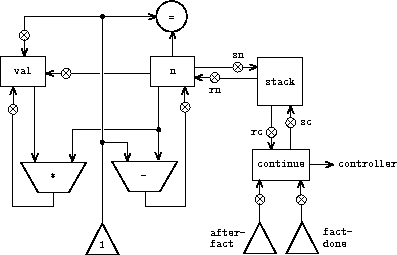(controller    (assign continue (label fact-done))     ; set up final return address  fact-loop    (test (op =) (reg n) (const 1))    (branch (label base-case))    ;; Set up for the recursive call by saving n and continue.    ;; Set up continue so that the computation will continue    ;; at after-fact when the subroutine returns.    (save continue)    (save n)    (assign n (op -) (reg n) (const 1))    (assign continue (label after-fact))    (goto (label fact-loop))  after-fact    (restore n)    (restore continue)    (assign val (op *) (reg n) (reg val))   ; val now contains n(n - 1)!    (goto (reg continue))                   ; return to caller  base-case    (assign val (const 1))                  ; base case: 1! = 1    (goto (reg continue))                   ; return to caller  fact-done)

 (controller    (assign continue (label fib-done))  fib-loop    (test (op <) (reg n) (const 2))    (branch (label immediate-answer))    ;; set up to compute Fib(n - 1)    (save continue)    (assign continue (label afterfib-n-1))    (save n)                           ; save old value of n    (assign n (op -) (reg n) (const 1)); clobber n to n - 1    (goto (label fib-loop))            ; perform recursive call  afterfib-n-1                         ; upon return, val contains Fib(n - 1)    (restore n)    (restore continue)    ;; set up to compute Fib(n - 2)    (assign n (op -) (reg n) (const 2))    (save continue)    (assign continue (label afterfib-n-2))    (save val)                         ; save Fib(n - 1)    (goto (label fib-loop))  afterfib-n-2                         ; upon return, val contains Fib(n - 2)    (assign n (reg val))               ; n now contains Fib(n - 2)    (restore val)                      ; val now contains Fib(n - 1)    (restore continue)    (assign val                        ;  Fib(n - 1) +  Fib(n - 2)            (op +) (reg val) (reg n))     (goto (reg continue))              ; return to caller, answer is in val  immediate-answer    (assign val (reg n))               ; base case:  Fib(n) = n    (goto (reg continue))  fib-done)

Exercise 5.4.  Specify register machines that implement each of the following procedures. For each machine, write a controller instruction sequence and draw a diagram showing the data paths.

a. Recursive exponentiation:

(define (expt b n)
(if (= n 0)
1
(* b (expt b (- n 1)))))

b. Iterative exponentiation:

(define (expt b n)
(define (expt-iter counter product)
(if (= counter 0)
product
(expt-iter (- counter 1) (* b product))))
(expt-iter n 1))

Exercise 5.5.  Hand-simulate the factorial and Fibonacci machines, using some nontrivial input (requiring execution of at least one recursive call). Show the contents of the stack at each significant point in the execution.

Exercise 5.6.  Ben Bitdiddle observes that the Fibonacci machine's controller sequence has an extra save and an extra restore, which can be removed to make a faster machine. Where are these instructions?

### 5.1.5  Instruction Summary

A controller instruction in our register-machine language has one of the following forms, where each <inputi> is either (reg <register-name>) or (const <constant-value>).

These instructions were introduced in section 5.1.1:

(assign <register-name> (reg <register-name>))

(assign <register-name> (const <constant-value>))

(assign <register-name> (op <operation-name>) <input1... <inputn>)

(perform (op <operation-name>) <input1... <inputn>)

(test (op <operation-name>) <input1... <inputn>)

(branch (label <label-name>))

(goto (label <label-name>))

The use of registers to hold labels was introduced in section 5.1.3:

(assign <register-name> (label <label-name>))

(goto (reg <register-name>))

Instructions to use the stack were introduced in section 5.1.4:

(save <register-name>)

(restore <register-name>)

The only kind of <constant-value> we have seen so far is a number, but later we will use strings, symbols, and lists. For example, (const "abc") is the string "abc", (const abc) is the symbol abc, (const (a b c)) is the list (a b c), and (const ()) is the empty list.

1 This assumption glosses over a great deal of complexity. Usually a large portion of the implementation of a Lisp system is dedicated to making reading and printing work.

2 One might argue that we don't need to save the old n; after we decrement it and solve the subproblem, we could simply increment it to recover the old value. Although this strategy works for factorial, it cannot work in general, since the old value of a register cannot always be computed from the new one.

3 In section 5.3 we will see how to implement a stack in terms of more primitive operations.